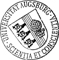## The average number of pivot steps of the simplex-algorithm based on a generalized rotation-symmetry-model

• This paper deals with the average-case-analysis of the number of pivot steps required by the simplex method. It generalizes results of Borgwardt (who worked under the assumpution of the rotation-symmetry-model) for the shadow-vertex-algorithm to so-called cylindric distributions. Simultaneously it allows to analyze an extended dimension-by-dimension-algorithm, which solves linear programing problems with arbitrary capacity bounds b in the restrictions Ax<=b, whereas the model used by Borgwardt required strictly positive right hand sides b. These extensions are achieved by solving a problem of stochastic geometry closely related to famous results of Renyi and Sulanke, namely: Assume that a_1,...,a_m are uniformly distributed in a cylinder. How many facets of conv(a_1,...,a_m,0) will be intersected by a two-dimensional shadow plane along the axis of the cylinder. The consequence of these investigations is that the upper bounds of Borgwardt (under his model) still apply when we acceptThis paper deals with the average-case-analysis of the number of pivot steps required by the simplex method. It generalizes results of Borgwardt (who worked under the assumpution of the rotation-symmetry-model) for the shadow-vertex-algorithm to so-called cylindric distributions. Simultaneously it allows to analyze an extended dimension-by-dimension-algorithm, which solves linear programing problems with arbitrary capacity bounds b in the restrictions Ax<=b, whereas the model used by Borgwardt required strictly positive right hand sides b. These extensions are achieved by solving a problem of stochastic geometry closely related to famous results of Renyi and Sulanke, namely: Assume that a_1,...,a_m are uniformly distributed in a cylinder. How many facets of conv(a_1,...,a_m,0) will be intersected by a two-dimensional shadow plane along the axis of the cylinder. The consequence of these investigations is that the upper bounds of Borgwardt (under his model) still apply when we accept distributions with arbitrary right hand sides.Author: Markus Göhl, Karl-Heinz BorgwardtGND urn:nbn:de:bvb:384-opus4-27649 https://opus.bibliothek.uni-augsburg.de/opus4/2764 Preprints des Instituts für Mathematik der Universität Augsburg (2014-04) Preprint English Universität Augsburg 2014/06/17 simplex method; shadow vertex algorithm; rotation symmetry model; cylinder distribution; average case analysis Simplexverfahren; Schatteneckenalgorithmus; Average-case-Komplexität `Auch erschienen in: Mathematical Methods of Operations Research, Volume 80, Issue 3 (2014),p. 329-366. DOI https://doi.org/10.1007/s00186-014-0483-8` Mathematisch-Naturwissenschaftlich-Technische Fakultät Mathematisch-Naturwissenschaftlich-Technische Fakultät / Institut für Mathematik Mathematisch-Naturwissenschaftlich-Technische Fakultät / Institut für Mathematik / Diskrete Mathematik, Optimierung und Operations Research 5 Naturwissenschaften und Mathematik / 51 Mathematik / 510 MathematikDeutsches Urheberrecht